# Four-vectors in Relativity

In the literature of relativity, space-time coordinates and the energy/momentum of a particle are often expressed in four-vector form. They are defined so that the length of a four-vector is invariant under a coordinate transformation. This invariance is associated with physical ideas. The invariance of the space-time four-vector is associated with the fact that the speed of light is a constant. The invariance of the energy-momentum four-vector is associated with the fact that the rest mass of a particle is invariant under coordinate transformations.

 The space-time 4-vector is defined by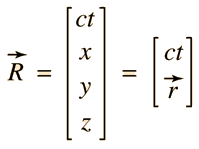The energy-momentum 4-vector is defined by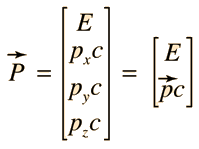The scalar product of two space-time 4-vectors is defined byand the scalar product of two energy-momentum 4-vectors by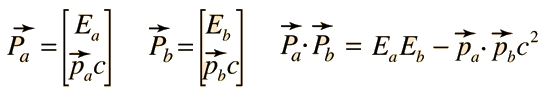Note that this differs from the ordinary scalar product of vectors because of the minus sign. That minus sign is necessary for the property of invariance of the length of the 4-vectors. The length of the space-time 4-vector squared is given by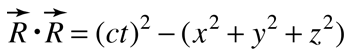The length of a 4-vector is invariant, being the same in every inertial frame. This invariance is associated with the constancy of the speed of light. This expression can be seen to be the equation of a sphere, with light propagating outward from the origin at speed c in all directions so that the radius of the sphere at time t is ct. The length of the energy-momentum 4-vector is given by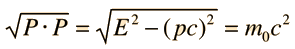The length of this 4-vector is the rest energy of the particle. The invariance is associated with the fact that the rest mass is the same in any inertial frame of reference.
 Lorentz transformation in 4-vector form
 Four-vector sum for momentum-energy
Index

Reference
Rohlf
Sec 4-4

 HyperPhysics***** Relativity R Nave
Go Back

# Lorentz Tranformation of Four-vectors

The Lorentz-transformation of both space-time and momentum-energy four-vectors can be expressed in matrix form.

 Space-Time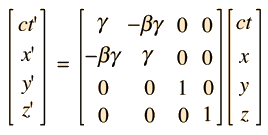Momentum-Energy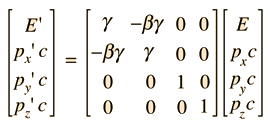The space-time Lorentz transformation produces the result: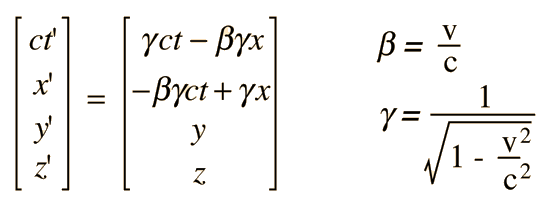and the energy-momentum 4-vector transforms as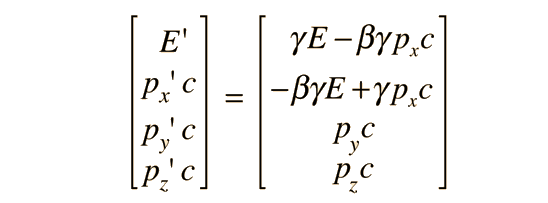Index

Reference
Rohlf
Sec 4-4

 HyperPhysics***** Relativity R Nave
Go Back

# Four-vector Sum for Momentum-Energy

Two momentum-energy four-vectors can be summed to form a four-vector.The length of this four-vector is an invariant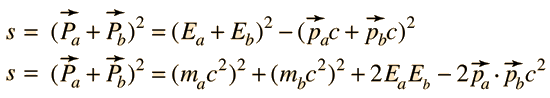The momenta of two particles in a collision can then be transformed into the zero-momentum frame for analysis, a significant advantage for high-energy collisions. For the two particles, you can determine the length of the momentum-energy 4-vector, which is an invariant under Lorentz transformation. The practical advantage of this for high energy collisions is that it allows you to calculate the momentum of each particle in the zero-momentum frame. One approach to this for a two particle system involves adding the momenta and energy for the two particles:Transforming this to the zero-momentum frameWhile this gives the form of the necessary transformation, we don't know the values for β and γ necessary to achieve the zero-momentum condition. That's where the invariance of the length of the energy-momentum 4-vector is of value. Now evaluating the length of the momentum-energy 4-vector from the experimental information we have in the laboratory frame gives the quantity s above. Since s can be evaluated from laboratory information, we can concentrate on the expression for s in the zero-momentum frame.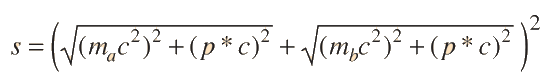where p*c has been used to represent the value of each momentum since they are constrained to be equal. This expression can be used to determine p*c, and that value can be compared to the original value of the momentum for one of the particles to determine the values of for β and γ necessary for the transformation.

 Example for photon-electron interaction
Index

Reference
Rohlf
Sec 4-4

 HyperPhysics***** Relativity R Nave
Go Back